# Selina Concise Mathematics Class 8 ICSE Solutions Chapter 12 Algebraic Identities

## Selina Concise Mathematics Class 8 ICSE Solutions Chapter 12 Algebraic Identities

Selina Publishers Concise Mathematics Class 8 ICSE Solutions Chapter 12 Algebraic Identities

### Algebraic Identities Exercise 12A – Selina Concise Mathematics Class 8 ICSE Solutions

Question 1.
Use direct method to evaluate the following products :
(i) (x + 8)(x + 3)
(ii) (y + 5)(y – 3)
(iii) (a – 8)(a + 2)
(iv) (b – 3)(b – 5)
(v) (3x – 2y)(2x + y)
(vi) (5a + 16)(3a – 7)
(vii) (8 – b) (3 + b)
Solution:Question 2.
Use direct method to evaluate :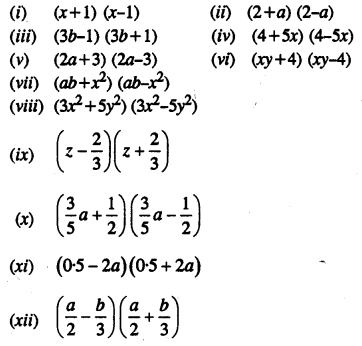Solution:Question 3.
Evaluate :Solution: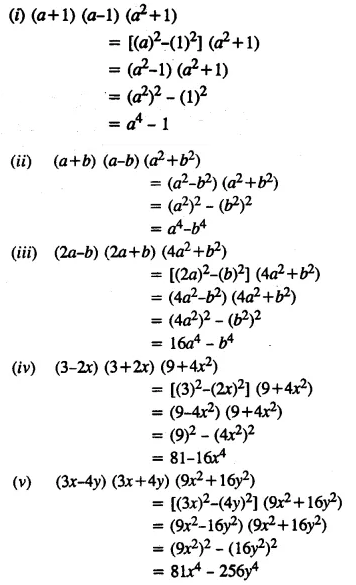Question 4.
Use the product (a + b) (a – b) = a2 – b2 to evaluate:
(i) 21 × 19
(ii) 33 × 27
(iii) 103 × 97
(iv) 9.8 × 10.2
(v) 7.7 × 8.3
(vi) 4.6 × 5.4
Solution: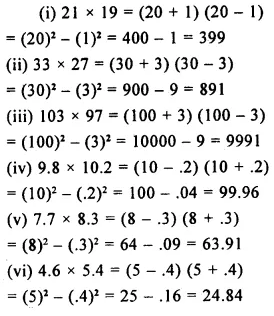Question 5.
Evaluate :
(i) (6 – xy) (6 + xy)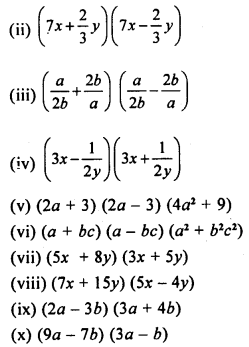Solution: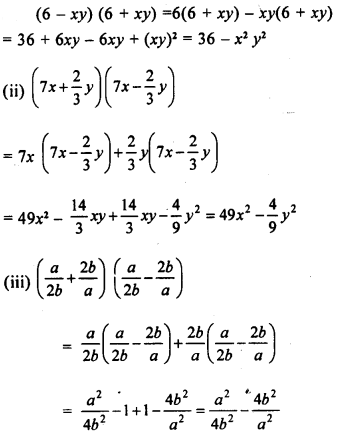### Algebraic Identities Exercise 12B – Selina Concise Mathematics Class 8 ICSE Solutions

Question 1.
Expand :
(i) (2a + b)2
(ii) (a – 2b)2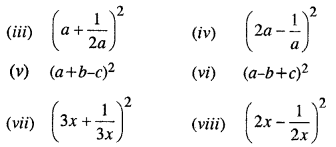Solution: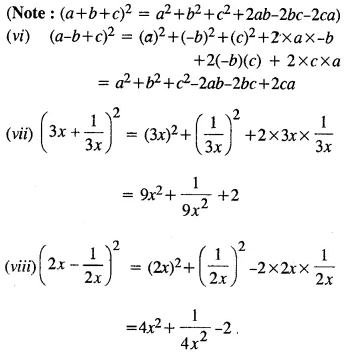Question 2.
Find the square of :Solution: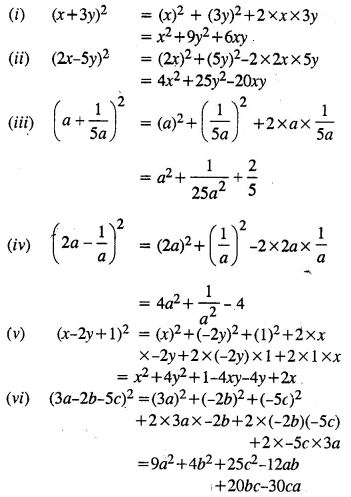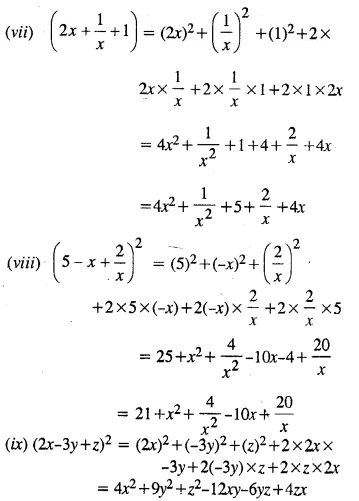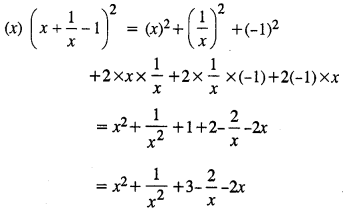Question 3.
Evaluate:
Using expansion of (a + b)2 or (a – b)2
(i) (208)2
(ii) (92)2
(iii)(415)2
(iv) (188)2
(v) (9.4)2
(vi) (20.7)2
Solution: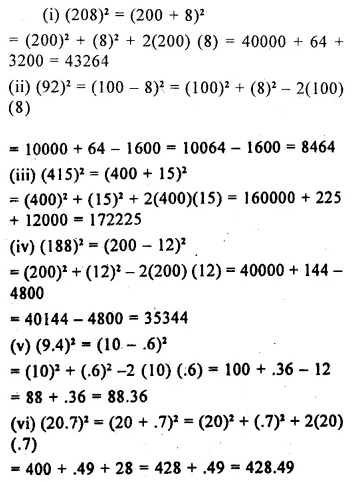Question 4.
Expand :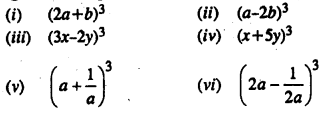Solution: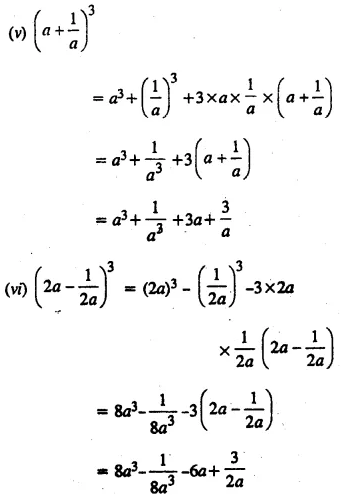Question 5.
Find the cube of :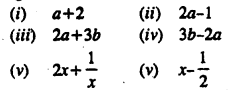Solution: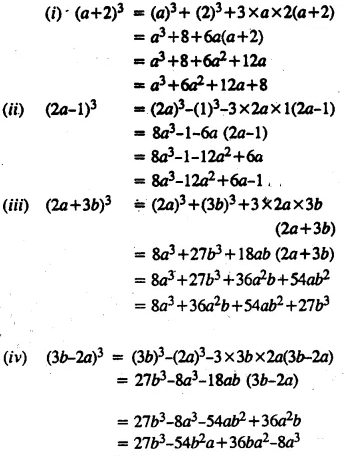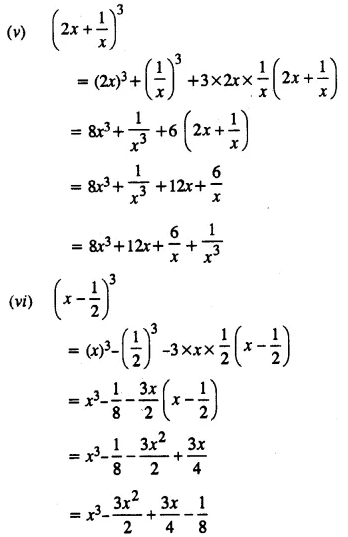### Algebraic Identities Exercise 12C – Selina Concise Mathematics Class 8 ICSE Solutions

Question 1.
If a  +b = 5 and ab = 6; find a2 + b2
Solution:Question 2.
If a – b = 6 and ab = 16; find a2 + b2
Solution: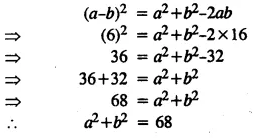Question 3.
If a2 + b2 = 29 and ab = 10 ; find :
(i) a + b
(ii) a – b
Solution: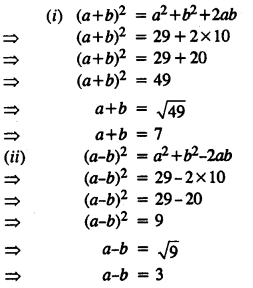Question 4.
If a2 + b= 10 and ab = 3; find :
(i) a – b
(ii) a + b
Solution:Question 5.Solution: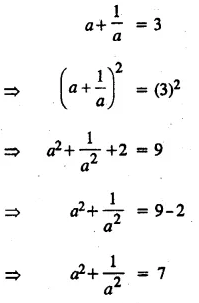Question 6.Solution: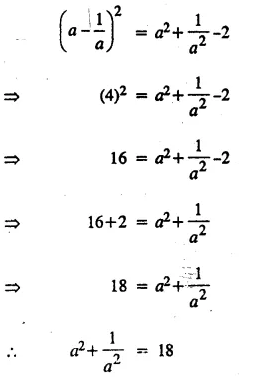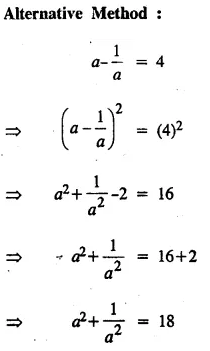Question 7.Solution:Question 8.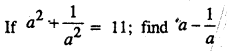Solution: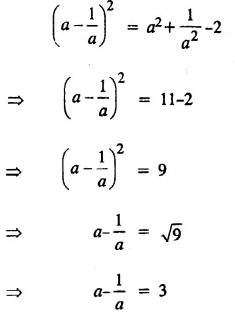Question 9.
If a + b + c = 10 and a2 + b2 + c2 = 38; find ab + bc + ca
Solution:Question 10.
Find a2 + b2 + c2 ; if a + b + c = 9 and ab + bc + ca = 24
Solution: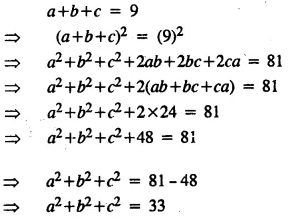Question 11.
Find a + b + c; if a2 + b2 + c2 = 83 and ab + bc + ca = 71
Solution:Question 12.
If a + b = 6 and ab=8; find a3 + b3
Solution: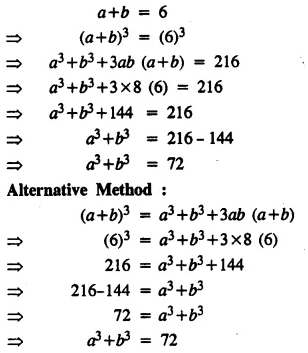Question 13.
If a – b = 3 and ab = 10; find a3 – b3
Solution: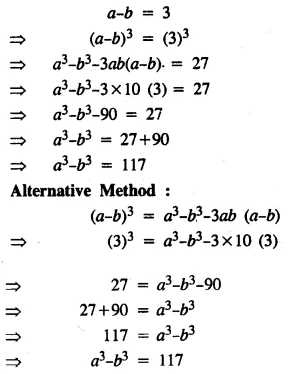Question 14.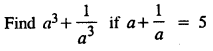Solution:Question 15.Solution: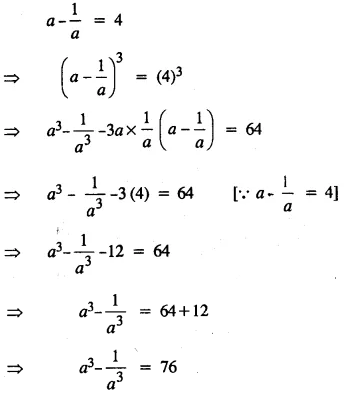Question 16.Solution: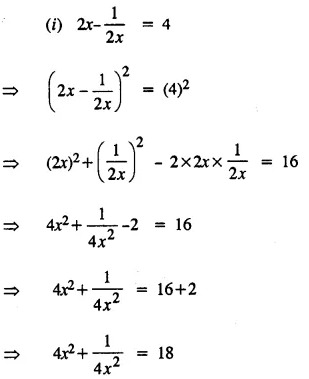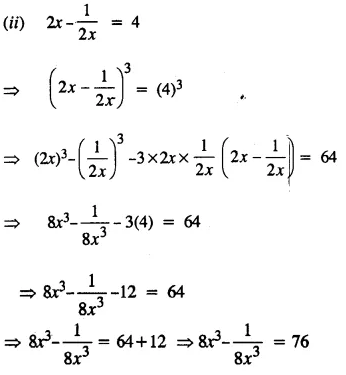Question 17.Solution: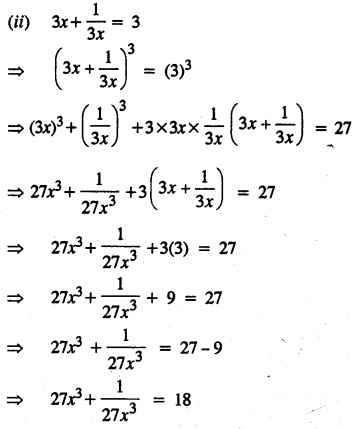Question 18.
The sum of the squares of two numbers is 13 and their product is 6. Find:
(i) the sum of the two numbers.
(ii) the difference between them.
Solution: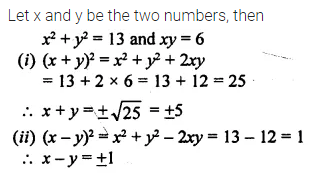### Algebraic Identities Exercise 12D – Selina Concise Mathematics Class 8 ICSE Solutions

Question 1.
Evaluate: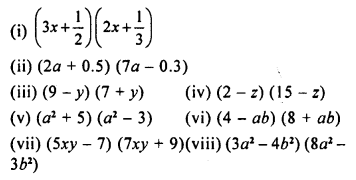Solution:Question 2.
Evaluate:Solution: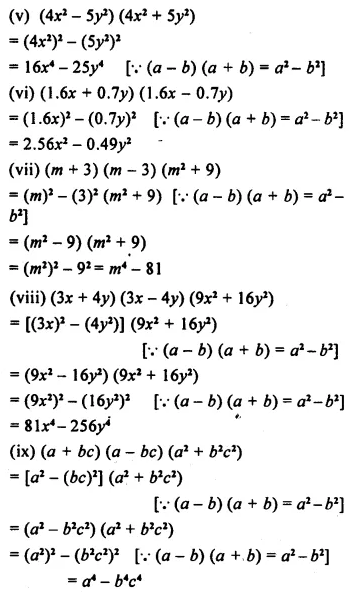Question 3.
Find the square of :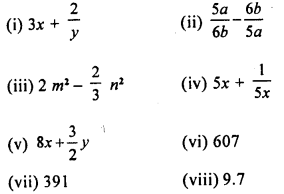Solution: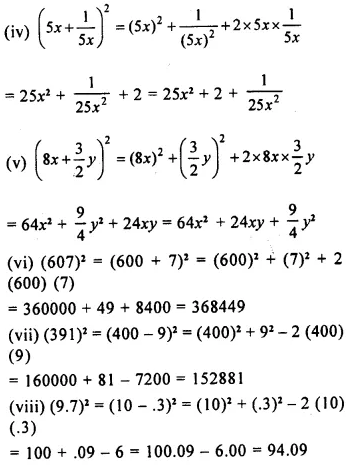Question 4.Solution:Question 5.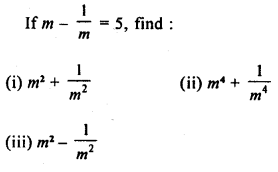Solution:Question 6.
If a2 + b2 = 41 and ab = 4, find :
(i) a – b
(ii) a + b
Solution: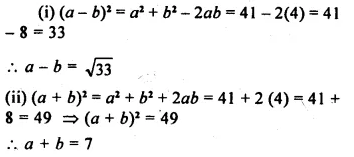Question 7.Solution:Question 8.Solution: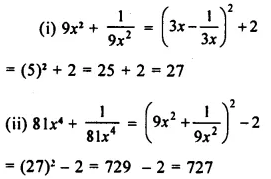Question 9.
Expand :
(i) (3x – 4y + 5z)2
(ii) (2a – 5b – 4c)2
(iii) (5x + 3y)3
(iv) (6a – 7b)3
Solution: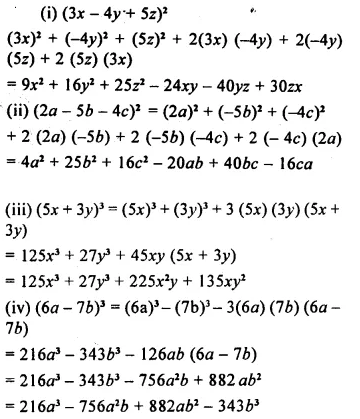Question 10.
If a + b + c = 9 and ab + bc + ca = 15, find: a2 + b2 + c2.
Solution:Question 11.
If a + b + c = 11 and a2 + b2 + c2 = 81, find ab + bc + ca.
Solution:Question 12.
If 3x – 4y = 5 and xy = 3, find : 27x3 – 64y3.
Solution: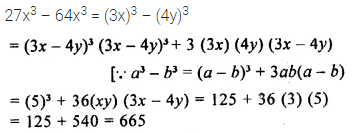Question 13.
If a + b = 8 and ab = 15, find : a3 + b3.
Solution: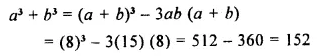Question 14.
If 3x + 2y = 9 and xy = 3, find : 27x3 + 8y3
Solution: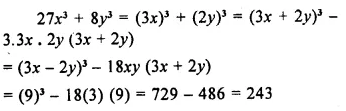Question 15.
If 5x – 4y = 7 and xy = 8, find : 125x3 – 64y3
Solution:Question 16.
The difference between two numbers is 5 and their products is 14. Find the difference between their cubes.
Solution: